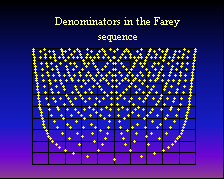## Discrete Optimisation and Combinatorial Geometry

#### Discrete Optimization and LatticesIn this research area we are interested in applications of Discrete Mathematics, especially Discrete Geometry and Minkowski's Geometry of Numbers, to a series of problems from Discrete Optimisation. The current research activities are focused on the geometry and combinatorics of lattice points in polyhedra, integer knapsack problems, lattice bases and integral generating sets. This research theme brings together researchers from Cardiff University, TU Berlin (Prof. Martin Henk), UC Davis (Prof. Jesus De Loera) and other institutions worldwide.

#### Cutting Planes in Integer Programming

This research direction focuses on improving cutting plane algorithms for general integer programs. This research area is developing in collaboration with Lancaster University (Prof. Adam Letchford).

#### Discrete Search and Asymptotic Distributions in Diophantine Approximations

As an example, consider the following group testing problem. Let a set of n factors is given and assume that only some of them are significant. Let T be the (unknown) group of significant factors. It is required to determine T by testing as few as possible factor groups. Assume that the result of the test for the factor group X can be written in the form f(X,T) = min {K, N(X,T)}, where N(X,T) is the number of elements in the intersection of X and T and K is an integer. (In binary screening problems K=1, in the multiaccess channel problem K=2 and in additive screening K=n.) To prove existence of a deterministic design of length N separating all factor groups it is enough to prove that the probability of separation of these groups is positive for a random design. Using this general principle suggested in an early work of A.Renyi, we can derive upper bounds for the length of optimal nonsequential group testing designs in different cases when the information about the number of elements in T and X is available. A general way of derivation of asymptotic upper bounds, when n tends to infinity, is also available. The technique is applied to many discrete search problems including searching with lies.

Another example deals with the accuracy of diophantine approximations expressed in probabilistic terms. Many diophantine approximation algorithms produce a sequence of sets F(n), indexed by n, of rational numbers p/q in [0,1]. Famous examples of F(n) are the Farey sequence, the collection of rationals p/q in [0,1] with q<=n, and the collection of all n-th continued fraction convergents. We consider several related accuracy characteristics of F(n) such as the average length of the uncertainty interval for a random x in [0,1], Renyi entropies of the partitions generated by the points in F(n), and the two-dimensional distribution of the distances between random x and both endpoints of the uncertainty interval. Properly normalized, these characteristics are expected to exhibit certain asymptotic behaviour when n tends to infinity. The corresponding results can typically be expressed in probabilistic language, in terms of weak convergence of sequences of probability measures if x is treated as a random point uniformly distributed on [0,1].

#### Cardiff Investigators

• Dr Iskander Aliev
• Prof Anatoly Zhigljavsky
• Prof Martin Huxley
• Dr Matthew Lettington

#### Collaborators

• Prof Jesus De Loera
• Dr Leonid Fukshansky
• Prof Martin Henk